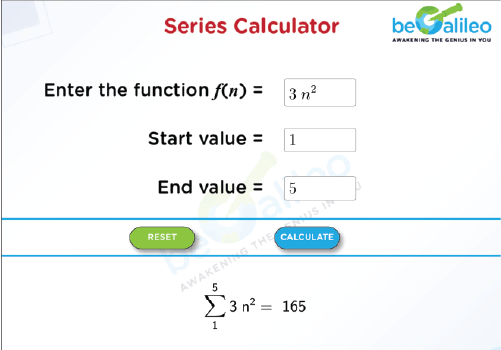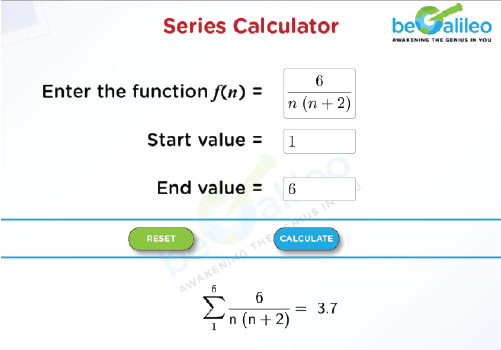>### Master Math with 1:1 Online Classes. Learn from Experts!# Series Calculator

A series calculator is a specialized computational tool or software designed to help users calculate the sum of mathematical series quickly and accurately. These calculators are particularly useful for handling complex or lengthy series, where manual calculations can become cumbersome or impractical.

(Note: A mathematical series is a sequence of numbers added together according to a specific pattern or formula)

## How to use the Series Calculator?

1. Function Entry : To start, enter the mathematical function you wish to sum. Make sure to represent it in terms of 'n'.
2. Range Specification : Enter the starting and ending values of ‘n’ in the corresponding input boxes to indicate the boundaries.
3. Calculation : Click "Calculate" to compute the summation once the function and range have been specified.
4. Result Display : The computed series for the given function will be displayed below the calculator.
5. Reset Option : If needed, you can reset the start and end values to their default settings by clicking the "Reset" button.

These steps will help you efficiently use the Series Calculator to determine the sum of your selected function.

## How to find the sum of a series?

Start by analyzing the function's mathematical expression or rule. For each input, compute the corresponding output value. This may involve substituting different input values into the function and calculating the resulting outputs. By doing this systematically, you can determine the full range of possible output values. After you have all the output numbers, add them up to find the total sum. That's your answer for the series you're looking at.

Example :
Let’s find the sum of the series of f(n) = 6n + 7 ; for n = 1, 2, 3, 4, 5.
We can start by simply calculating the value of the function for each ‘n’ and then add up the results.

For n = 1 : f(1) = 6(1) + 7 = 6 + 7 = 13
For n = 2 : f(2) = 6(2) + 7 = 12 + 7 = 19
For n = 3 : f(3) = 6(3) + 7 = 18 + 7 = 25
For n = 4 : f(4) = 6(4) + 7 = 24 + 7 = 31
For n = 5 : f(5) = 6(5) + 7 = 30 + 7 = 37

13 + 19 + 25 + 31 + 37 = 125

So, the sum of the series f(n) = 6n + 7 for n = 1, 2, 3, 4, 5 is 125.

## Solved Examples

### Q1. Find the sum of the series for f(n)=3^2 for n = 1, 2, 3, 4, 5.

Solution :
f(1)=3(1)^2=3\times1=3
f(2)=3(2)^2=3\times4=12
f(3)=3(3)^2=3\times9=27
f(4)=3(4)^2=3\times16=48
f(5)=3(5)^2=3\times25=75
Sum of the series: 3 + 12 + 27 + 48 + 75 = 165
So, the sum of the series f(n)=3(n)^2 for n = 1, 2, 3, 4, 5 is 165.### Q2. Find the sum of the series for f(n)=\frac{6}{n(n+2)} for n = 1, 2, 3, 4, 5, 6.

Solution :
f(1)=\frac{6}{1(1+2)}=\frac{6}{3}=2
f(2)=\frac{6}{2(2+2)}=\frac{6}{8}=\frac{3}{4}
f(3)=\frac{6}{3(3+2)}=\frac{6}{15}=\frac{2}{5}
f(4)=\frac{6}{4(4+2)}=\frac{6}{24}=\frac{1}{4}
f(5)=\frac{6}{5(5+2)}=\frac{6}{35}
f(6)=\frac{6}{6(6+2)}=\frac{6}{48}=\frac{1}{8}
Sum of the series: 2+\frac{3}{4}+\frac{2}{5}+\frac{1}{4}+\frac{6}{35}+\frac{1}{8}=\frac{207}{56}\asymp 3.7
So, the sum of the series f(n)=\frac{6}{n(n+2)}for n = 1, 2, 3, 4, 5, 6 is 3.7.